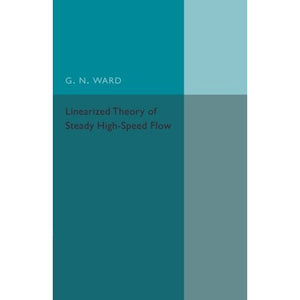># Linearized Theory of Steady High-Speed Flow

• £14.19
• Save £14

G. N. Ward
Cambridge University Press, 3/3/2016
EAN 9781316601891, ISBN10: 1316601897

Paperback, 260 pages, 21.6 x 14 x 1.7 cm
Language: English

The difficulty of solving the non-linear equations of motion for compressible fluids has caused the linear approximations to these equations to be used extensively in applications to aeronautics. Originally published in 1955, this book is the first permanent work devoted exclusively to the problems involved in this important and rapidly developing subject. The first part of the book gives the derivation and interpretation of the linear equations for steady motion, the solution of these equations and a discussion of the boundary conditions and aerodynamic forces. The remainder examines various specific boundary value problems and the methods, which have been developed for their solution. Vectorial notation is used extensively throughout and an elementary familiarity with the theory and practice of compressible fluid flow is required. This book will be of considerable value to scholars of physics and mathematics as well as to anyone with an interest in the history of education.

Preface
Part I. General Theory
1. Linearization of the equations of motion
2. The general solutions of the linearized equations for subsonic flow
3. The general solutions of the linearized equations for supersonic flow
4. Boundary conditions, aerodynamic forces, uniqueness and flow-reversal theorems
Part II. Special Methods
5. Subsonic flow past thin bodies
6. Supersonic flow past nearly plane wings
7. Conical fields in supersonic flow
8. Application of operational methods to supersonic flow
Part III. Slender-Body Theory
9. Flow at and near the surfaces of slender bodies
Appendix 1. Integral identities
Appendix 2. The linearized equations for nearly parallel steady flows
Bibliography
Index.